﻿ 基于S波段新一代天气雷达观测的下击暴流临近预报方法
 快速检索 中文标题 英文标题 作者中文名 作者英文名 单位中文名 单位英文名 中文关键词 英文关键词 中文摘要 英文摘要 基金项目 全文
 气象2021, Vol. 47Issue (8): 919-931.  DOI: 10.7519/j.issn.1000-0526.2021.08.002### 引用本文 [复制中英文]

[复制中文]
XIAO Yanjiao, WANG Jue, WANG Zhibin, et al, 2021. A Downburst Nowcasting Method Based on Observations of S-Band New Generation Weather Radar[J]. Meteorological Monthly, 47(8): 919-931. DOI: 10.7519/j.issn.1000-0526.2021.08.002.
[复制英文]

### 文章历史

2020年8月10日收稿
2021年3月23日收修定稿

A Downburst Nowcasting Method Based on Observations of S-Band New Generation Weather Radar
, WANG Jue, WANG Zhibin, LENG Liang, FU Zhikang
Hubei Key Laboratory for Heavy Rain Monitoring and Warning Research, Institute of Heavy Rain, CMA, Wuhan 430205
Abstract: Downburst is the most common weather phenomenon of convective storm, and forecasting its initial outbreak is one of the most challenging contents in severe convective storm forecasting. In this paper, a downburst nowcasting algorithm based on radar and radiosonde observation data was proposed. On the basis of ground clutter suppression and radial velocity dealiasing of radar base data and processing of sounding data to obtain 0℃, -20℃ and minimum equivalent potential temperature heights, the algorithm first identifies and tracks storm cells and calculates the hail indexes, then identifies the mid-level radial convergence characteristics and mesocyclone, making them associated with the identified storm cell. After that, many radar characteristics of storm cells are extracted. After statistical analysis of downburst and non-downburst cases, nine radar precursor factors of downburst were selected as the input of fuzzy logic method, and the nowcasting equation of downburst was established. The algorithm was tested with a downburst case which occurred in Jianli of Hubei Province on 1 June 2015, responsible for the "Oriental Star" cruise ship capsizing. The results show that the algorithm has predicted 8 times in 20:41-21:21 BT that the storm cell having caused the shipwreck will produce downburst. The first prediction time is 47 minutes earlier than that of the cruise ship capsizing at 21:28 BT. In addition, the effectiveness of the downburst nowcasting algorithm was evaluated by using all thunderstorm gales in Hubei Province from June to August 2019. The results show that the hit rate of downburst is 86.4%, and the average forecast time is 39 min. According to the echo pattern, the hit rates of downburst nowcasting for squall line, linear convection and non-linear convection are 93.2%, 90.5% and 75.6%, respectively. Actually, the algorithm module has been integrated into the automatic identification and warning system of classified severe convective weather developed by Wuhan Institute of Heavy Rain of CMA, and has been put into operation since 2019. The algorithm will be continuously optimized in the forecasting operation application in the future.
Key words: downburst    nowcasting algorithm    weather radar

Fujita(1979)根据国际上第一次针对下击暴流开展的外场观测计划(the Northern Illinois Meteorological Research on Downburst)观测到的50个下击暴流的统计特征，正式定义了下击暴流：雷暴天气中在地面或近地面附近产生风速＞17.9 m·s-1的灾害性辐散风的强下沉气流。Fujita(1985)按下击暴流地面出流的灾害范围大小，把下击暴流分为宏下击暴流(＞4 km)和微下击暴流(≤4 km)。Wilson et al(1984)基于多普勒雷达的观测对微下击暴流进行了补充定义：穿过辐散中心的多普勒速度差≥10 m·s-1，初始的最大入流和出流中心(正负速度对)之间的距离＜4 km。Hjelmfelt(1988)把下击暴流按出流形态分为孤立的微下击暴流和下击暴流线。

1 资料和预处理

2 下击暴流临近预报方法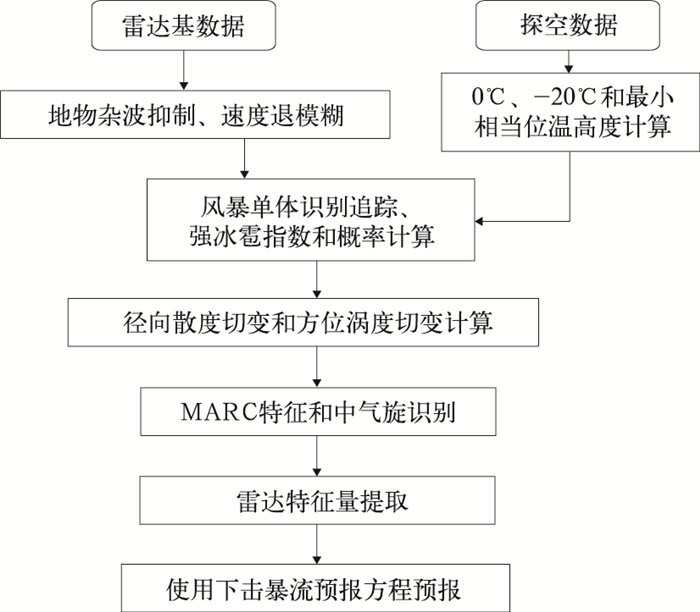图 1 下击暴流临近预报算法流程图 Fig. 1 Flow chart of downburst nowcasting algorithm
2.1 风暴单体识别、追踪和冰雹指数计算

 $S H I=0.1 \int_{H_{0}}^{H_{\mathrm{T}}} W_{\mathrm{T}}(H) E \mathrm{~d} H$ (1)
 $P O S H=29 \ln \left(\frac{S H I}{W T}\right)+50$ (2)

 $E=5 \times 10^{-6} \times 10^{0.084 Z} W(Z)$ (3)
 $W_{\mathrm{T}}(H)= \begin{cases}0 & H \leqslant H_{0} \\ \frac{H-H_{0}}{H_{\mathrm{m} 20}-H_{0}} & H_{0} $ W T=57.5 H_{0}-121 $(5) $ W(Z)=\left\{\begin{array}{lc} 0 & Z \leqslant Z_{\mathrm{L}} \\ \frac{Z-Z_{\mathrm{L}}}{Z_{\mathrm{U}}-Z_{\mathrm{L}}} & Z_{\mathrm{L}}

E为冰雹动能通量，单位为J·m-2·s-1WT(H)为基于温度的权重函数，W(Z)为用于定义降雨和冰雹反射率因子转换区的权重函数，WT为与零度层高度有关的冰雹警报阈值，单位为J·m-1·s-1

2.2 径向散度和方位涡度切变估计

 $\begin{gathered} u_{\mathrm{r}}=\frac{\sum\limits_{j} \sum\limits_{i} i u_{i, j} w_{i, j}}{\Delta r \sum\limits_{j} \sum\limits_{i} i^{2} w_{i, j}} \\ (i=-N, \cdots, 0, 1, \cdots, N ; j=-M, \cdots, 0, 1, \cdots, M) \end{gathered}$ (7)
 $\begin{gathered} u_{\mathrm{a}}=\frac{\sum\limits_{j} \sum\limits_{i} S_{i, j} u_{i, j} w_{i, j}}{\sum\limits_{j} \sum\limits_{i} S_{i, j}{ }^{2} w_{i, j}} \\ (i=-N, \cdots, 0, 1, \cdots, N ; j=-M, \cdots, 0, 1, \cdots, M) \end{gathered}$ (8)

 $N=(\text { int })\left[\left(\frac{D}{\Delta r}-1\right) / 2\right]$ (9)
 $M=(\text { int })\left[\left(\frac{D}{r \Delta \varphi}-1\right) / 2\right]$ (10)

2.3 MARC特征和中气旋识别

(1) 一维切变段识别

(2) 二维切变特征识别

(3) 三维切变特征识别

2.4 风暴单体雷达特征参量提取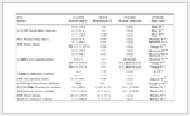表 1 用于下击暴流临近预报的风暴单体雷达特征量隶属函数的高、低临界值和权重 Table 1 The high and low critical values and weights of the membership function of P=\frac{\sum_{i=1}^{n} F\left(x_{i}\right) W\left(x_{i}\right)}{\sum_{i=1}^{n} W\left(x_{i}\right)}
2.5 下击暴流临近预报方程建立

 $F(x)=\left\{\begin{array}{lc} 0 & x \leqslant T_{\mathrm{L}} \\ \frac{x-T_{L}}{T_{\mathrm{U}}-T_{\mathrm{L}}} & T_{\mathrm{L}} 把雷达特征量作为输入变量，使用模糊逻辑法建立下击暴流临近预报概率方程： $ P=\frac{\sum\limits_{i=1}^{n} F\left(x_{i}\right) W\left(x_{i}\right)}{\sum\limits_{i=1}^{n} W\left(x_{i}\right)} \$ (12)

3 个例分析

2015年6月1日21:28(北京时，下同)左右，“东方之星”客轮在长江航道湖北监利段开始侧翻，21:31倾覆，导致442人死亡。两个气象专家组进行了现场调查和多种探测资料综合分析，得出相似结论。孟智勇等(2016)研究表明，客轮在倾覆时处于一条飑线弓形回波顶点处，根据树木折断情况估计遭遇了至少31 m·s-1的强风，该强风可能是由微下击暴流里的直线大风或其中的小尺度涡旋(非龙卷)造成。郑永光等(2016)研究表明，客轮倾覆江段和周边区域发生了显著微下击暴流导致的强烈大风灾害，估计最强风力超过12级，并具有空间分布不连续、多尺度和强灾害时空尺度小等特征。事发周边区域北部的四台村养猪场附近树林中同时发生了多条相邻的微下击暴流条迹，呈现出辐散和辐合交替分布的特征。由于微下击暴流尺度非常小，现有地面自动气象观测站网没有捕捉到这次微下击暴流大风过程。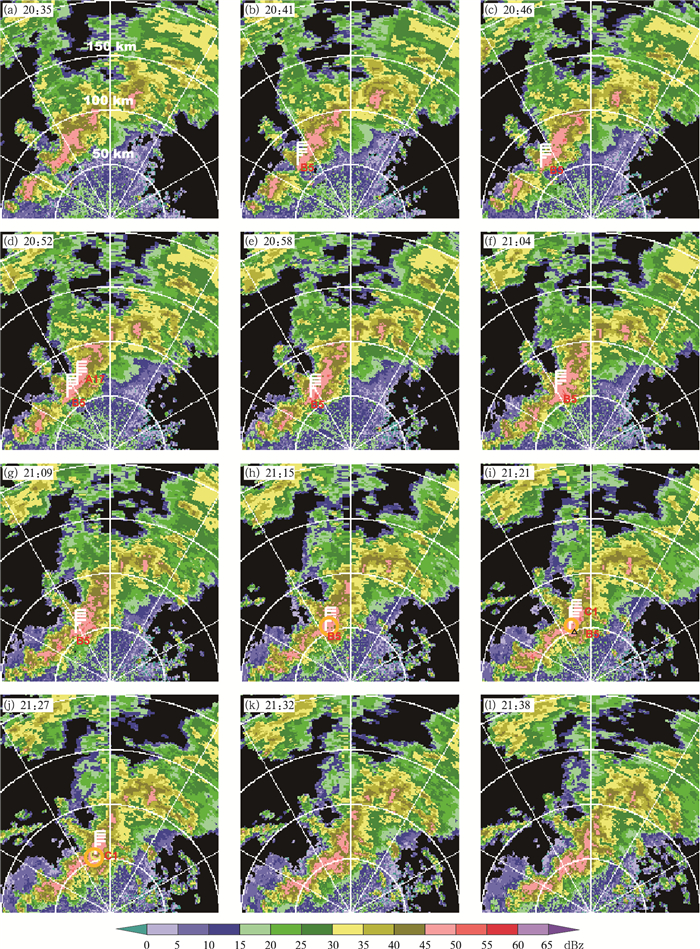图 2 2015年6月1日20:35-21:38基于岳阳雷达的下击暴流预报结果叠加0.5°仰角反射率因子 (图中字母是预报会产生下击暴流的风暴, 白色风杆不表示风向和风速，只表示该风暴单体将会产生下击暴流，风杆的南端点是MARC质心位置; 黄色圆圈是识别的中等强度中气旋，图2i中的空心三角形所在处为客轮倾覆地点) Fig. 2 Downburst forecast results superimposed on the 0.5° elevation reflectivity factor map at 20:35-21:38 BT 1 June 2015 based on Yueyang Radar (Letters represent the storm predicted to produce downburst; white wind pole does not indicate the wind direction and speed, but the storm cell to produce downburst; the southern end of wind pole is the position of MARC centroid, yellow circle is the identified moderate intensity mesocyclone, and hollow triangle in Fig. 2i is the capsizing point of the passenger ship)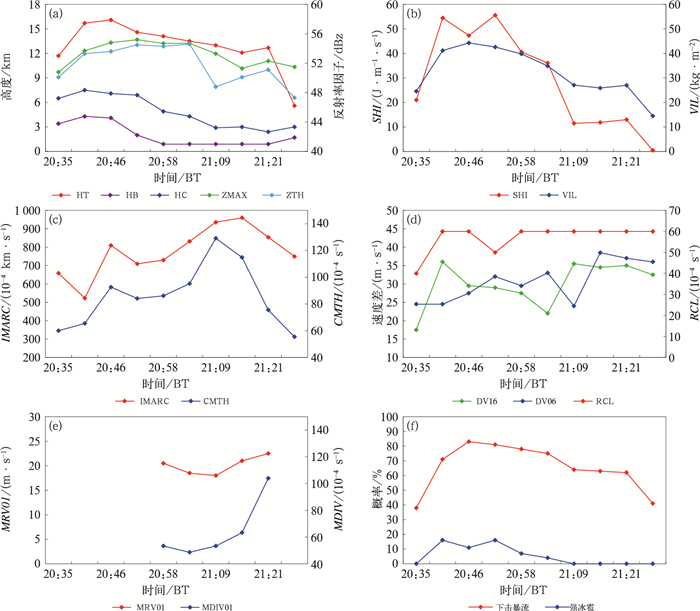图 3 2015年6月1日导致“东方之星”客轮倾覆的风暴单体的(a~e)雷达特征量、(f)预报的下击暴流和强冰雹的概率的时间序列 (a)HT、HB、HC、ZMAX和ZTH, (b)VIL和SHI, (c) IMARC和CMTH, (d)DV16、DV06和RCL, (e) MRV01和MDIV01 Fig. 3 Time series of radar characteristics, (a-e) predicted downburst and (f) severe hail probability of the storm cell triggering the capsizing of "Oriental Star" Passenger Ship on 1 June 2015 (a) HT, HB, HC, ZMAX, and ZTH, (b) VIL and SHI, (c) IMARC and CMTH, (d) DV16, DV06 and RCL, (e) MRV01 and MDIV01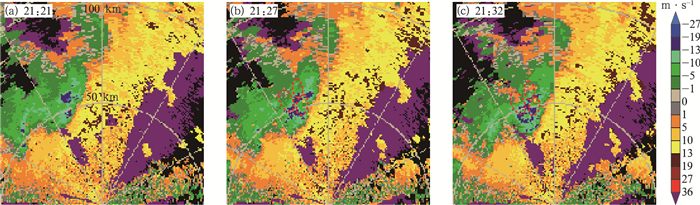图 4 2015年6月1日(a)21:21，(b)21：27，(c)21:32岳阳雷达观测的0.5°仰角径向速度 (图中红色椭圆为径向辐散区) Fig. 4 Radial velocity at 0.5° elevation observed by Yueyang Radar at (a) 21:21 BT, (b) 21:27 BT, (c) 21:32 BT 1 June 2015 (The red ellipses are the radial divergence areas)
4 预报效果评估表 2 用于评估的雷暴大风天气个例 Table 2 Thunderstorm and gale weather cases for assessment

5 结论与讨论

(1) 综合使用雷达反射率因子、径向速度和基于探空观测的0℃、-20℃和最小相当位温高度等资料，基于风暴单体识别追踪、冰雹指数计算、MARC特征识别和中气旋识别等算法，提取风暴单体的诸多雷达特征量，经统计分析挑选出下击暴流的雷达先兆因子9个作为模糊逻辑法的输入，建立了下击暴流临近预报方程。

(2) 使用2015年6月1日发生在监利导致“东方之星”客轮侧翻的下击暴流个例对本算法进行了测试，结果表明本算法从20:41-21:21共有8个体扫时次预报了引起沉船事件的那个风暴单体将会产生下击暴流，首次预报时间比客轮侧翻时间早47 min。21:27-21:32，0.5°仰角的径向速度中出现了辐散式的正负速度对，表明下击暴流大风的发生。

(3) 使用2019年6-8月发生在湖北省的雷暴大风个例对本文提出的下击暴流临近预报算法进行了效果评估，结果表明本算法对下击暴流临近预报的击中率为86.4%，平均预报时效为39 min；按回波形态分类评估，飑线类、线状对流类(飑线除外)和非线状对流类风暴的下击暴流临近预报击中率分别为93.2%、90.5%和75.6%。